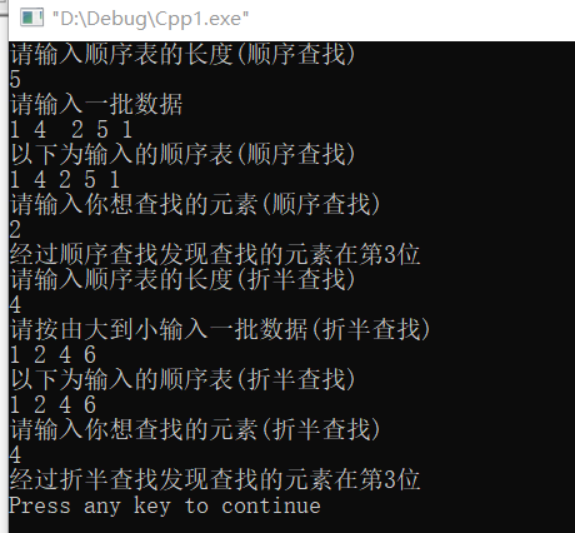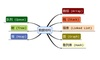• 1.建立两个顺序表（通过随机函数生成）； 2.排序（升序），输出合并前的结果； 3.对这两个顺序表进行合并（保持升序）； 4.输出合并结果
• *顺序表结构体里面包含 数据数组 和 数组长度 *采用两种查找方法 哨兵设置 普通查找 *哨兵排序算法的设置的好处是可以降低时间的复杂度 节省 for循环的次数 *程序 的步骤分为 初始化顺序表 创建顺序表 查找 输出 ...
/* *顺序表的查找算法（重点是哨兵的设置） *创建一个数据结构体 *创建一个顺序表的结构体 *顺序表结构体里面包含 数据数组 和 数组长度 *采用两种查找方法 哨兵设置 普通查找 *哨兵排序算法的设置的好处是可以降低时间的复杂度 节省 for循环的次数 *程序 的步骤分为 初始化顺序表 创建顺序表 查找 输出 测试 */
#include<stdio.h>
#include<stdlib.h>
#include<string.h>
#define MAX_SIZE 1024//数据的个数
typedef  int KeyType;//关键字的数据类型
typedef  char* ValueType;//标识字符串的数据类型
typedef int Statu;//返回值的数据类型

#define ERROR 0
#define OK 1
typedef struct elementtype
{
KeyType key;//关键字
ValueType value;//数据域

}ElementType;//顺序表中的数据结构体
typedef struct seqlist
{
ElementType *datas;//数据数组
int length;//顺序表长度
}SeqList;//顺序表点的结构体数据类型
void InIt(SeqList*s);//顺序表的初始化
void test();//测试函数
void print_seqlist(SeqList*s);//打印顺序表
Statu search_seqlist1(SeqList*s,KeyType key);//顺序表的节点数据的查找
Statu search_seqlist2(SeqList*s,KeyType key);//顺序表的节点数据的查找(设置哨兵元素)

void main()
{
test();//测试函数
}
void InIt(SeqList*s)//顺序表的初始化
{
s->datas=(ElementType*)malloc(sizeof(ElementType)*MAX_SIZE);//创建顺序表的储存空间
s->length=0;//初始化顺序表的长度
}
void print_seqlist(SeqList*s)//打印顺序表的元素
{
int i;
for(i=1;i<=s->length;i++)//下标为0的位置不放数据
printf("%d %s\n",s->datas[i].key,s->datas[i].value);

}
Statu search_seqlist1(SeqList*s,KeyType key)//顺序表的节点数据的查找
{
int i;
// printf("%d\n",s->length);
for(i=1;i<=s->length;i++)
{
if(s->datas[i].key==key)
return i;
}
return 0;
}
Statu search_seqlist2(SeqList*s,KeyType key)//顺序表的节点数据的查找(设置哨兵元素)
{
//好处节省了for循环中循环条件的判断
//设置哨兵和将第一个数组的位置空下来是一样的
s->datas.key=key;
int i;
for(i=s->length-1;s->datas[i].key!=key;i--)
return i;

}


展开全文• 实验六 查找 //顺序查找和二分查找 #include "stdio.h" #include "stdlib.h" #define N 20 typedef struct { int Key; }ElemType; typedef struct SSTable { ElemType *elem; int length; }SSTable; int Search_Seq...
• 题目 已知一组数据，采用顺序存储结构存储，其中所有的元素为整数。设计一个算法，删除元素值在[x,y]之间的所有元素 #include &lt;stdio.h&gt; #define infinity 0.173 int main() {  int sum,i,j = ...
题目 已知一组数据，采用顺序存储结构存储，其中所有的元素为整数。设计一个算法，删除元素值在[x,y]之间的所有元素#include <stdio.h> #define infinity 0.173 int main() {    int sum,i,j = 0,k;    int min,max;     scanf("%d",&sum);     int a[sum];     float t[sum];          for(i = 0;i < sum;i++)        t[i] = infinity;                     for(i = 0;i < sum;i++)         scanf("%d",&a[i]);              scanf("%d%d",&min,&max);        for(i = 0;i < sum;i++){        if(a[i] >= min && a[i] <= max)         continue;          t[j] = a[i];          j++;    }        k = j;    for(i = 0;i < k;i++){        if(i != k - 1)          printf("%.0f ",t[i]);         else{             printf("%.0f",t[i]);             break;         }    }     return 0; }
展开全文• C语言顺序表顺序查找和折半查找的基本思想和应用 顺序查找算法：又称为线性查找，主要用在—线性表—中进行查找 通常分为：1—无序线性表的一般查找； 2—对关键字有序的顺序表查找； 优缺点分析： 缺点：当线性表的...
C语言顺序表顺序查找和折半查找的基本思想和应用
顺序查找算法：又称为线性查找，主要用在—线性表—中进行查找 通常分为：1—无序线性表的一般查找； 2—对关键字有序的顺序表查找； 优缺点分析： 缺点：当线性表的表长过于长时，平均查找长度较大，效率低。 优点：对顺序表中数据元素的存储没有要求，顺序存储链式存储均可。 需注意：对于线性表的链式存储只能使用顺序查找. 折半查找，又称二分查找，它仅适用于有序的顺序表 首先将给定值key与表中间位置元素的关键字比较，若相等，则查找成功，返回该元素的存储位置；(例如，在查找表升序排列时，若给定值key大于中间元素的关键字，则所查找的关键字只可能在后半部分)若不等，则所需查找的元素只能在中间元素以外的前半部分或后半部分中；然后在缩小范围内继续进行同样的查找，如此重复直到找到为止，若查找不成功，返回查找失败信息 优缺点分析： 折半查找方法的优点：折半查找的优点是比较次数少，查找速度快，平均性能好； 折半查找方法的缺点：折半查找的缺点是：要求待查表为有序表，且插入删除困难。 因此：折半查找方法适用于不经常变动而查找频繁的有序列表。
目的是：⑴ 输入一批整型数据，建立顺序表，然后用顺序查找； ⑵ 输入一批有序整型数据（如从小到大），然后用折半查找，查找一给定的整数。
#include <stdlib.h>
#define maxsize 20//全局定义

#define OK 1
#define OVERFLOW -2
typedef struct
{
int key;//关键字
}ElemType;
typedef struct
{
ElemType *elem;
int length;
}sqlist;
int initList(sqlist &L)
{
L.elem=new ElemType[maxsize];//分配数组存储空间
if(!L.elem) exit (OVERFLOW);
L.length=0;
return OK;
}
void display(sqlist &L)//自定义输出函数，将顺序表的key值输出。
{
int i;
for(i=0;i<L.length;i++)
printf("%d ",L.elem[i].key);
printf("\n");
}
void get(sqlist &L,int size)//3.自定义取值函数利用循环依次为elem[i].key取值，
{
for(int i=0;i<size;i++)
{
scanf("%d",&L.elem[i].key);
L.length++;
}
}
int search(sqlist L,int key)//自定义顺序查找函数，在顺序表L中顺序查找其关键字等于key的数据元素，若找到，则函数值为该元素在表中的位置，否则为0.
{
for(int i=L.length;i>=1;--i)
if(L.elem[i].key==key)  return i;
return 0;
}
int search_Bin(sqlist L,int key)//6.自定义折半查找函数，置查找区间初值，low为1，high为表长；当low小于等于high时，mid取low和high的中间值，将给定值key与中间位置记录的关键字进行比较，若查找成功返回mid，若不相等则利用中间位置记录将表对分成前后两个子表。如果key比中间位置记录的关键字小，则high取为mid-1,否则low取为mid+1；循环结束，说明查找区间为空，则查找失败，返回0.
{
int low=1,high=L.length,mid;
while(low<=high)
{
mid=(low+high)/2;
if(key==L.elem[mid].key) return mid;
else if(key<L.elem[mid].key)  high=mid-1;
else  low=mid+1;
}
return 0;
}

int main()
{
int m,x,y;
int m1,x1,y1;
sqlist L,M;
initList(L);
initList(M);
printf("请输入顺序表的长度(顺序查找)\n");
scanf("%d",&m);
printf("请输入一批数据\n");
get(L,m);
printf("以下为输入的顺序表(顺序查找)\n");
display(L);
printf("请输入你想查找的元素(顺序查找)\n");
scanf("%d",&x);
y=search(L,x);
printf("经过顺序查找发现查找的元素在第%d位\n",y+1);
printf("请输入顺序表的长度(折半查找)\n");
scanf("%d",&m1);
printf("请按由大到小输入一批数据(折半查找)\n");
get(M,m1);
printf("以下为输入的顺序表(折半查找)\n");
display(M);
printf("请输入你想查找的元素(折半查找)\n");
scanf("%d",&x1);
y1=search_Bin(M,x1);
printf("经过折半查找发现查找的元素在第%d位\n",y1+1);
return 0;

}C语言数据结构第二版（严蔚敏、李冬梅）著

展开全文• *顺序表的相关操作 *创建顺序表（顺序存储） *创建数组 ...*遍历顺序表数据 */ #include<stdio.h> #include<time.h> #include<stdlib.h> #include<string.h> #define MAXSI...

*顺序表的相关操作 *创建顺序表（顺序存储） *创建数组 *在指定位置进行删除 *在指定位置进行插入 *返回指定位置的数据 *返回数据的指定位置 *遍历顺序表的数据 */
#include<stdio.h>
#include<time.h>
#include<stdlib.h>
#include<string.h>
#define  MAXSIZE 1024//顺序表的数据最大数目
#define NAME_SIZE 255//字符串的最大长度
#define ERROR 0
#define OK 1
typedef  int Statu;//函数返回值的数据类型
typedef  char *NameTye;//数据名称的数据类型
typedef int IdType;//数据的编号的数据类型
typedef struct elementtype
{
NameTye  name;//数据的名称
IdType id;//数据的编号
}ElementType;//数据域的结构体类型

typedef struct Seq_List
{
ElementType *data;//顺序表指针进行动态赋储存空间
int last;//指向数据下标的指针（类似指针）

}Seq_List;//顺序表的结构体类型
void Init_seqlist(Seq_List*s);//对顺序表进行初始化
Statu create_seqlist(Seq_List*s);//对顺序表进行创建
int delet_position(Seq_List*s,int i);//在指定位置进行删除
int inset_seqlist(Seq_List*s,int i,ElementType key);//在指定位置进行插入
int search_seqlist(Seq_List*s,ElementType key);//返回数据的位置（不是下标）
int  search_data_seqlist(Seq_List*s,int i,ElementType *x);//返回指定位置的数据
void Display_seqlist(Seq_List*s);//对顺序表进行遍历
void sort_seqlist(Seq_List*s);//对顺序表进行排序
int value_length_seqlist(Seq_List*s);//计算顺序表的长度
void main()
{
int i;
int index;//要删除数据元素的位置
int index2;//要插入的位置
Seq_List*s;//顺序表指针
int index3;//要返回数据位置的下标
ElementType key;//要插入的数据
ElementType str;//要查询的数据
ElementType x;
s=(Seq_List*)malloc(sizeof(Seq_List));  //对其进行初始化

Init_seqlist(s);//对顺序表进行创建

int result=create_seqlist(s);
if(result==ERROR)
{
printf("顺序表创建失败:\n");
}
else
{
printf("顺序表创建成功:\n");
printf("顺序表遍历的结果为:\n");
Display_seqlist(s);
}
printf("\n");
printf("顺序表的长度为%d\n",value_length_seqlist(s));
printf("对顺序表中的元素排序的结果为:\n");
sort_seqlist(s);
for(i=0;i<s->last+1;i++)
printf("[%s,%d]->",s->data[i].name,s->data[i].id);
printf("\n");//换行
printf("输入要删除的数据的位置:\n");
scanf("%d",&index);
int result1=delet_position(s,index);
if(result1==ERROR)
printf("删除指定节点失败:\n");
else
{
printf("删除指点位置的节点成功:\n");
printf("删除之后数据的遍历结果为:\n");
Display_seqlist(s);
printf("\n");
}
printf("请输入要插入的节点的名称:\n");
key.name=(NameTye)malloc(sizeof(char)*NAME_SIZE);
key.id=rand()%50;//产生随机数字
scanf("%s",key.name);
printf("请输入要插入的位置:\n");
scanf("%d",&index2);
int result2=inset_seqlist(s,index2,key);
if(result2==ERROR)
{
printf("在指定位置插入数据失败:\n");
}
else
{
printf("在指定位置插入节点成功\n");
printf("插入数据之后的遍历的结果为\n");
Display_seqlist(s);
printf("\n");
}
printf("清输入要查找的数据:\n");
str.name=(NameTye)malloc(sizeof(char)*NAME_SIZE);
str.id=rand()%50;//产生随机数字
//对数据名称指针进行动态赋存储空间
scanf("%s",str.name);
int pos=search_seqlist(s,str);
if(pos==ERROR)
{
printf("查询数据失败:\n");
}
else{
printf("查询数据成功\n");
printf("要查询的数据在顺序表中的位置是%d\n",pos);
}
printf("请输入要返回数据位置：\n");
x.name=(NameTye)malloc(sizeof(char)*NAME_SIZE);
//进行动态分配存储空间 让其能存储字符串
scanf("%d",&index3);
int result3=search_data_seqlist(s,index3,&x);
if(result3==ERROR)
{
printf("该数据在顺序表中不存在:\n");

}
else
{

printf("查找数据成功\n");
printf("在顺序表位置为%d的数据为[%s,%d]",index3,x.name,x.id);
}

}
void Init_seqlist(Seq_List*s)
{
s->data=(ElementType*)malloc(sizeof(ElementType)*MAXSIZE);
//对顺序表的数组进行动态赋存储空间
s->last=-1;//last的位置是数据元素在数组中的下标
}
Statu create_seqlist(Seq_List*s)//对顺序表进行创建
{
int i=0;//数组的循环变量
if(s==NULL)
{
return ERROR;//创建失败
}

char str[NAME_SIZE];
printf("请输入数据的名称:\n");
while(scanf("%s",str)!=EOF)
{
if(i+1==MAXSIZE)
break;//跳出while循环
if(strcmp(str,"^")==0)
break;//跳出循环
else
{
s->data[i].name=(NameTye)malloc(sizeof(char)*NAME_SIZE);
strcpy(s->data[i].name,str);
s->data[i].id=rand()%50;//产生随机数据
i++;
s->last++;//将顺序表的指针进行自加

}
}
return OK;
}
void  Display_seqlist(Seq_List*s)
{
int i;
if(s==NULL)
printf("链表为空，遍历失败！\n");
else
{
for(i=0;i<s->last+1;i++)
{
printf("[%s,%d]->",s->data[i].name,s->data[i].id);

}
}

}
int value_length_seqlist(Seq_List*s)//计算顺序表的长度
{
return (s->last+1);//顺序表的长度为s->last的长度加一
}
void sort_seqlist(Seq_List*s)//对顺序表进行排序
{
int i=s->last;//需要比较的次数
int j;
int flag=1;
ElementType *t;
t=(ElementType*)malloc(sizeof(ElementType));
while(flag)
{
flag=0;//判断是否继续进行循环的操作
for(j=0;j<i;j++)
{
if(s->data[j].id>s->data[j+1].id)
{
//递增排序
*t=s->data[j];
s->data[j]=s->data[j+1];
s->data[j+1]=*t;//交换数据
flag=1;//循环继续进行标志
}
}
i--;//循环次数减一
}
}
int delet_position(Seq_List*s,int i)//在指定位置进行删除
{
int j;//循环变量
if(s->last==-1)
{
return ERROR;//顺序表为空  删除失败
}
else if(i<=0)
{
return ERROR;//删除位置违法
}

else
{
for(j=i-1;j<=s->last;j++)
{
s->data[j]=s->data[j+1];
}
s->last--;//顺序表的节点个数减一
return OK;
}
}
int inset_seqlist(Seq_List*s,int i,ElementType key)//在指定位置进行插入
{
int j;//循环变量
if(s->last+1>=MAXSIZE)
{
return ERROR;
}
else if(i<=0)
{
return ERROR;
}
else
{
for(j=s->last;j>=i-1;j--)
{
s->data[j+1]=s->data[j];
}
s->data[i-1]=key;
s->last++;//last指针加一
return OK;//插入成功
}
}
int search_seqlist(Seq_List*s,ElementType key)//返回数据的位置（不是下标）
{
int i;//循环变量
for(i=0;i<s->last+1;i++)
{
if(strcmp(s->data[i].name,key.name)==0)
{
break;
}
}
return (i==s->last+1 ? ERROR : i+1 );
}
int  search_data_seqlist(Seq_List*s,int i,ElementType *x)//返回指定位置的数据
{
int j;
//对数据的位置进行判断
if(i<=0)//位置违法
return ERROR;
else if(s->last==-1)
return ERROR;//顺序表为空
else
{
x->id=s->data[i-1].id;
strcpy(x->name,s->data[i-1].name);//对字符串进行赋值
return OK;//返回数据成功
}
}


展开全文• /* 当前存储空间已满,增加分配 */ newbase=(ElemType *)realloc(L.elem,(L.listsize+LISTINCREMENT)*sizeof(ElemType)); 这句怎么改为用new表达？ newbase=new //怎么写这句c 存储
•线性表
• C语言数据结构顺序表的增删改查 tips：前些天学习了链表的操作以及相关的数据结构，今天我们来学习一下c语言数据结构顺序表的增删改查。 顺序表采用顺序存储，即把逻辑上相邻的元素存储在物理位置上也相邻的...算法 链表
• c语言数据结构顺序表： 顺序表的结构跟数组比起来还是很像的，相比于链表，数据表的优势主要体现在他的查询速度上，而链表的优势相反，查询速度慢，但对于插入一个数据来说还是比较快的下面我们就来创建一个顺序...
• C语言实现 顺序表 Init(初始化) Length(当前结点个数) Add(添加) Insert(插入) Delete(删除) FindByKey(查找返回序号) FindByNum(查找返回元素) ShowAll(显示所有元素)
• //C语言--数据结构--线性表 顺序表 线性表的顺序存储结构 //其实顺序表就相当于一个数组 #include #include #include #include #include using namespace std; #define MAXSIZE 10//定义顺序表的长度 ...线性表 线性表的顺序存储结构
• 顺序表：即在计算机中以数组结构保存的线性表，与数组类似，但可以对其进行插入删除等操作，其中数据类型是一样的，并且存储在一个连续的区域内。下面创建顺序表并进行测试；#include <stdio.h>#define ListSize 100...线性表
• 说来惭愧由于贪玩，数据结构挂科了，现在重新学一遍数据结构，...listsize代表这个顺序表的最大容量 可以随时扩容 length代表表中元素个数 应小于listsize 1.初始化 Status list_init(SqList &L) { L.elem...
• (数据结构C语言版)顺序表和单链表的逆置.docx文档
• ## C语言数据结构学生成绩 顺序表

千次阅读 多人点赞 2019-04-10 22:37:37
一个简单的顺序表实现一组学生信息增，删，查，取功能。其中文件导入部分的处理花费了较多时间 代码如下： 在这里插入代码片 #include<stdio.h> #include<stdlib.h> #include<string.h> #...线性表
• 数据结构-基于C语言实现线性顺序表的增删改查+排序，合表操作
• 算法与数据结构 C语言描述 创建顺序表并循环存入数据，自己手打的，希望大家支持！
• 数据结构C语言完成顺序表基本操作，上数据结构课的时候的任务，可以在vs上实现，用switch函数选择
• 顺序表顺序储存结构C语言实现版 #include <stdio.h> #define MAXSIZE 100 #define OVERFLOW 0 #define OK 1 #define ERROR 0 int main(){ } //顺序表顺序储存结构 ，这里泛型定义为整形 typedef struct{ ...
• 顺序表是一种简单的数据存储结构，既然把数据存起来就肯定要用，利用顺序表充当集合来实现集合的常用3种运算是一种常见的问题。代码如下： #include&lt;stdio.h&gt; #include&lt;stdlib.h&gt; #...集合运算
• 顺序表 按顺序存储的线性表叫顺序表（array - based list），通过创建数组来建立。顺序表的的元素是紧挨着的，见下图： 0 1 2 3 4 5 6 7 8 9 26 48 32 13 53 62 43 26 11 86 这个线性表的表长为10，即储存...线性表
• 本篇推文主要给大家介绍顺序表插入和删除的操作原理以及其具体的代码实现，了解顺序表的几种基本运算，要注意理解插入删除算法的原理掌握其实现代码 顺序表的基本运算 顺序表的插入运算 顺序表上完成插入运算的步骤...
• 问题：试写一算法，实现顺序表的就地逆置，即利用原表的存储空间将线性表(a1,a2,...,an)逆置为(an,an-1,...,a1) 算法思想：观察原表和新表顺序，逆置后的线性表是将原表相应位置的元素进行交换，即交换第一个和...
• 第2章 线性表 本章主要介绍下列内容 线性表的逻辑结构 线性表的顺序存储结构 线性表的链式存储结构 线性表的应用举例 2.1 线性表的逻辑结构 2.1.1 线性表的定义 线性表是由nn0个类型相同的数据元素组成的有限序列......

# c语言数据结构顺序表c语言 订阅数据结构 订阅